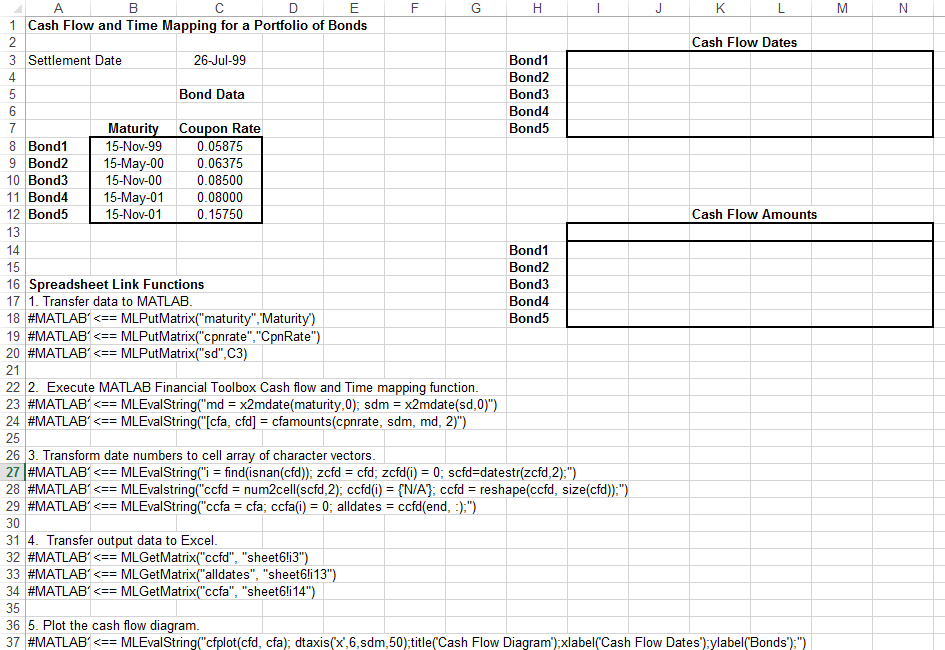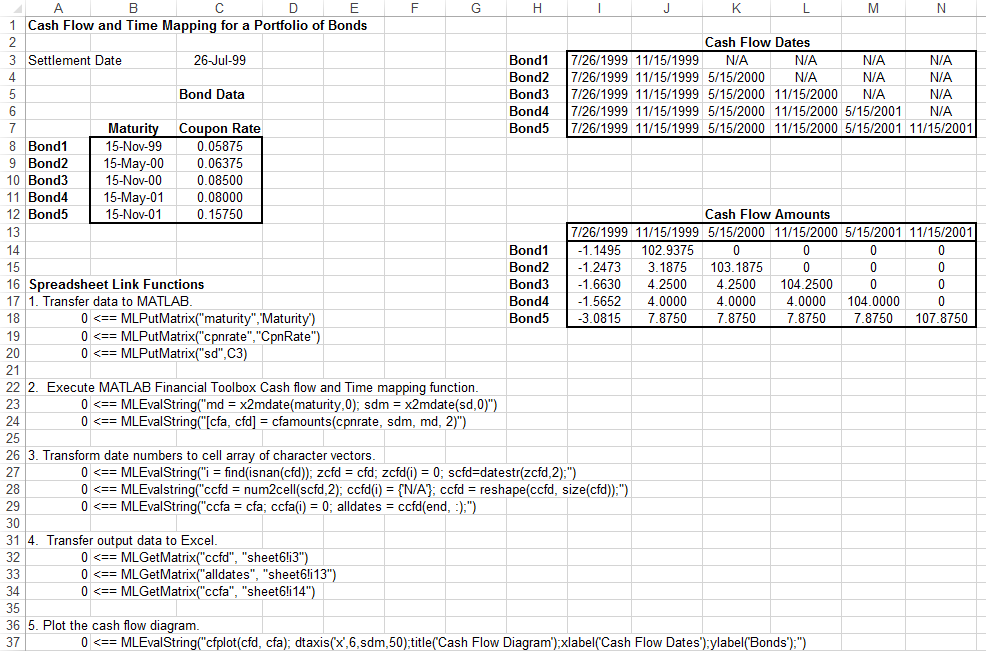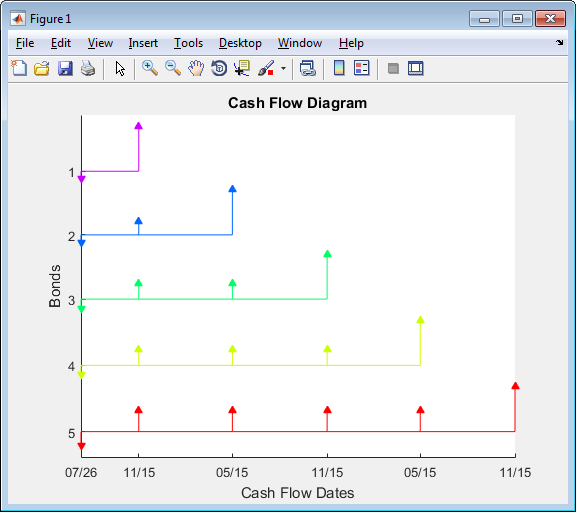## Map Time and Bond Cash Flows

This example shows how to use Financial Toolbox™ and Spreadsheet Link™ to calculate a set of cash flow amounts and dates for a portfolio of five bonds.

Open the `ExliSamp.xls` file and select the Sheet6 worksheet. For help finding the `ExliSamp.xls` file, see Installation.

This worksheet contains the maturity dates and coupon rates for five bonds.### Note

This example requires Financial Toolbox, Statistics and Machine Learning Toolbox™, and Optimization Toolbox™.

1. Execute the Spreadsheet Link function that transfers the column vector `Maturity` to the MATLAB® workspace by double-clicking the cell `A18` and pressing Enter.

2. Transfer the column vector `Coupon Rate` to the MATLAB workspace by executing the function in cell `A19`.

3. Transfer the settlement date to the MATLAB workspace by executing the function in cell `A20`.

4. Calculate cash flow amounts and dates by executing the Financial Toolbox functions in cells `A23` and `A24`.

5. Transform the dates into a cell array of character vectors by executing the functions in cells `A27` through `A29`.

6. Transfer the data to the Excel® worksheet by executing the functions in cells `A32` through `A34`.7. Display a plot of cash flows for each bond by executing the function in cell `A37`.To generate cash flows for a different set of five bonds, close the figure and change the bond data in cells `B8:C12`. Then, execute all the Spreadsheet Link functions again. The worksheet updates with new cash flow dates and amounts and MATLAB generates a new figure of the cash flows.